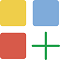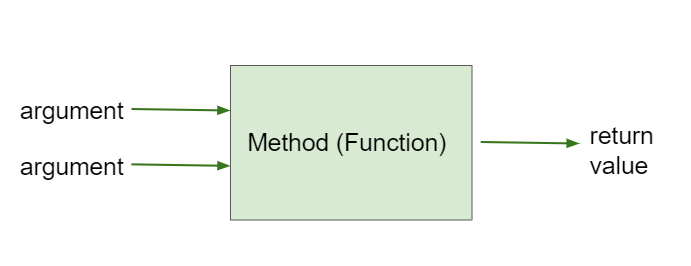# 2.5. Calling Methods that Return Values¶

If a method is a void method and has void as its return type, like most of the methods we have seen so far, that means that it does not return anything. But some methods return a value back that the program can use.

## 2.5.1. Get Methods¶

Get methods return the value of instance variables, for example getWidth() and getHeight() to get the height and width for a Turtle object.

In the previous lesson, we used some set methods with parameters to set the attributes of a turtle to different values, for example yertle.setColor(Color.red); or yertle.setWidth(50); Programmers create get and set methods for each attribute represented as an instance variable in a class to access and modify the value in that variable. The get methods always return back the value of that instance variable, and the set methods modify the value.

When you use a get method, you need to save what it returns in a variable or use the value in some way for example by printing it out. The data type of the variable must match the data type of the return value of the method. You can find out the return type of a method in its documentation. It will be right before the method name, for example int getWidth() means getWidth will return an int (an integer number).

Here are some examples of using get methods for the turtle object yertle.

```Turtle yertle = new Turtle(world);
int width = yertle.getWidth();
int height = yertle.getHeight();
System.out.println("Yertle's width is: " + width);
System.out.println("Yertle's height is: " + height);
System.out.println("Yertle's x position is: " + yertle.getXPos() );
System.out.println("Yertle's y position is: " + yertle.getYPos() );
```

Note

A common error is forgetting to do something with the value returned from a method. When you call a method that returns a value, you should do something with that value like saving it into a variable or printing it out.Coding Exercise:

Try the code below that changes the turtle’s width and height. How big or small can you make yertle?

(If the code below does not work in your browser, you can also copy in the code below into the Turtle code at this repl.it link (refresh page after forking and if it gets stuck) or download the files here to use in your own IDE.)Coding Exercise:

Fix the errors in the code below so that it prints out the area of the space that the turtle occupies by multiplying its width and height. Remember that you have to do something with the values that the get methods return.

## 2.5.2. toString() Methods¶

Another common method that returns a value is the toString() method. This method is called automatically to try to convert an object to a String when it is needed, for example in a print statement. In the Turtle class, the toString() method returns a String description of the turtle.

```Turtle yertle = new Turtle(world);
yertle.setName("yertle"); // set name before you use toString()
System.out.println(yertle.toString());
// Or you can just use the object here and it will call toString() automatically!
System.out.println(yertle);
```Coding Exercise:

Try some of the get methods and the toString() method in the program below. Note that you have to print out what the get methods return in order to see what they do!

## 2.5.3. Methods with Arguments and Return Values¶

Methods that take arguments and return values are like mathematical functions. Given some input, they return a value. For example, a square(x) method would take an argument x and return its square by multiplying it by itself.Figure 1: Method that takes arguments and returns a value¶

You will not write your own methods until Unit 5, but you should be able to trace through method calls like below. Notice that the return statement in a method returns the value that is indicated in the return type back to the calling method. The calling method must save or use or print that value.Check your understanding

Try this visualization to see this code in action.

## 2.5.4.Programming Challenge : Turtle Distances¶

1. The Turtle class has a method called getDistance(x,y) which will return the turtle’s distance from a point (x,y). Can you find yertle’s distance from the point (0,0)?

2. Add another turtle and make both turtles move. Then find the distance between them. You must use the getXPos() and getYPos() methods as well as the getDistance() method.

## 2.5.5. Summary¶

• Some methods return values.

• To use the return value when calling a method, it must be stored in a variable or used as part of an expression. The variable data type must match the return type of the method.

## 2.5.6. AP Practice¶

You have attempted of activities on this page